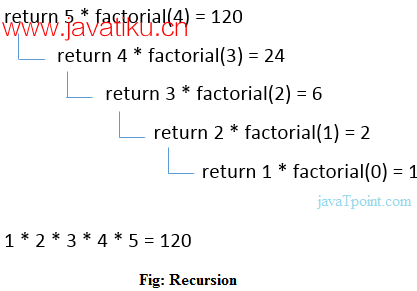# C++教程-C++递归## C++递归

``````cppCopy code
void recursionfunction() {
recursionfunction(); // 调用自身的函数
}``````

### C++递归示例

``````cppCopy code
#include<iostream>
using namespace std;

int factorial(int);

int main() {
int value, fact;
cout << "Enter any number: ";
cin >> value;
fact = factorial(value);
cout << "Factorial of a number is: " << fact << endl;
return 0;
}

int factorial(int n) {
if (n < 0)
return (-1); /* 错误的值 */
if (n == 0)
return (1); /* 终止条件 */
else {
return (n * factorial(n - 1));
}
}``````

``````Enter any number: 5
Factorial of a number is: 120``````